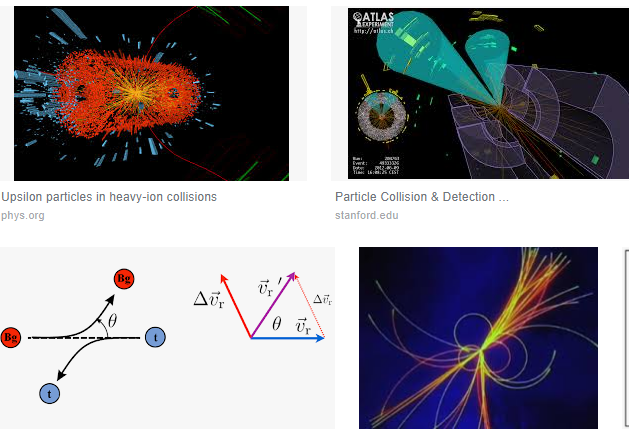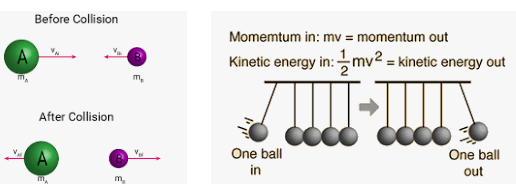Describe the collision between particles?

Collision between particles is defined as : 1. The mutual interaction of the particle for a small interval of time due to which both the energy and momentum of the interacting particle change.2. There are so many examples of collision from our day to day life e.g., collision between two gaseous atoms at room temperature.

3. It is not necessary for two bodies undergoing collision to have physical with contact with each other. In case of Rutherford’s α-particle scattering experiment, α-particles were scattered due to electrostatic interaction between α-particle and the nucleus separated by some distance.
Hence, in Physics a collision will take place if either of the two bodies comes in physical contact with each other or even when path of one body is affected by the force exerted due to the other.

A) Elastic Collision between particles:

The collisions, in which both the momentum and kinetic energy of the system remain conserved, are called elastic collision between particles e.g. the collision between atomic and subatomic particles, the collision between two glass balls etc.Elastic Collision between particles

Following characteristics are found in elastic collisions between particles:

a) The momentum is conserved
b) Total energy is conserved
c) Kinetic energy is conserved
d) Forces involved in the interaction are of conservative nature
e) Mechanical energy is not transformed into any other form of energy.

B) Inelastic Collision between particles:

The collisions in which only the momentum of the system is conserved but kinetic energy is not conserved are called inelastic collisions between particles. Most of the collisions in our day to day life are inelastic collisions. E.g., if a ball is dropped from a certain height and the balls unable to rise completely to its original height, then it would mean that ball has lost some kinetic energy. This would mean that collision is inelastic.Inelastic Collision between particles
Following characteristic are found in inelastic collisions:

a) Momentum is conserved
b) Total energy is conserved
c) Kinetic energy is not conserved
d) Some or all the forces involved are non-conservative in nature
e) A part or whole of the mechanical energy may be transformed into other forms of energy.

C) Perfectly inelastic or plastic collision: If the two bodies stick together after the collision, the collision is said to be perfectly inelastic. In this type of collision loss of kinetic energy is maximum but not complete. E.g. mud thrown on the wall is an example of perfectly inelastic collision. There is a complete loss of kinetic energy, as the mud remains sticking to the wall.5. Thus we find that in both type of collision,
a) Momentum is conserved
b) Total energy is conserved
c) It is the kinetic energy which may or may not be conserved.